nextnano.com  GUI: nextnanomat  Tool: nextnano++  Tool: nextnano³  Tool: nextnano.NEGF  Download | Search | Copyright | News | Publications  * password protected nextnano³ softwareGeometry objects region

Regions

Basic geometry objects

To built up a geometry, there are - dependent on the dimension of the simulation to be performed - various basic geometry elements available.
These geometry elements are specified within the keyword \$regions and can be clustered to a bigger object later on.

General specifiers

region-number =

an integer number >= 1
Numbering must be unique. All region numbers together must form a dense set 1,2,3,....,maxnumber

region-priority =

an integer number >= 1
In case of overlapping regions, the region with higher priority (= higher numerical value) overwrites the region with lower priority.

!--------------------------------------------------!
\$regions                                optional   !
region-number       integer            required   ! an integer number to refer to geometry element
region-priority     integer            required   ! a positive integer to set overwriting priority, a smaller number
! can be overwitten by any higher
base-geometry       character          required   ! type of geometry object:
! 3D: cuboid, sphere, ...

!
2D: rectangle, circle, ...

!
1D: line
x-coordinates       double_array       optional   ! for cuboid, rectangle, line: xmin, xmax
y-coordinates       double_array       optional   ! for cuboid, rectangle, line: ymin, ymax
z-coordinates       double_array       optional   ! for cuboid, rectangle, line: zmin, zmax
!
center              double_array       optional   !
for circle (2D), sphere (3D)
radius              double             optional   ! for circle (2D), sphere (3D)
!
base-coordinates    double_array       optional   !
for obelisk, cone, truncated-cone, trapezoid:

! xmin xmax ymin ymax zmin zmax
of obelisk (cone) base plane
! (one pair must be equal)
top-coordinates     double_array       optional   ! for obelisk, cone, truncated-cone, trapezoid, semiellipsoid:
! xmin xmax ymin ymax zmin zmax of obelisk (cone) base plane
! (one pair must be equal)
! x y z
for semiellipsoid
!
corner-coordinates  double_array       optional   !
for triangle:  x1 y1  x2 y2  x3y3
! triangle corner coordinates, interpreted via orientation and similar for polygon
!
semi-ellipse-base   double_array       optional   !
for semiellipse: base line ellipse:
! two pairs of xmin,xmax ymin,ymax zmin,zmax - one pair equal numbers
semi-ellipse-top    double_array       optional   ! for semiellipse: top coordinate pair: e.g. for coordinate orientation  (101) -> (x,z)

\$end_regions                            optional   !
!--------------------------------------------------!

• line

2-dimensional objects (only possible in 2D simulations):

• rectangle
• circle
• triangle
• trapezoid
• polygon
• regular-polygon    (not implemented yet but could be added ==> use polygon instead)
• hexagon            (not implemented yet but could be added ==> use polygon instead)
• semiellipse

3-dimensional objects (only possible in 3D simulations):

• cuboid
• sphere
• cylinder           (not implemented yet but could be added ==> use truncated-cone instead)
• obelisk
• hexagonal-obelisk
• cone
• truncated-cone
• semiellipsoid
• regular-prism      (not implemented yet but could be added)
• hexagonal-prism    (not implemented yet but could be added ==> use hexagonal-obelisk instead)
• triangular-prism
• polygonal-prism    (not implemented yet but could be added)
• pyramid            (not implemented yet but could be added ==> use obelisk instead)
• regular-pyramid    (not implemented yet but could be added ==> use obelisk instead)
• hexagonal-pyramid  (not implemented yet but could be added ==> use hexagonal-obelisk instead)
• polygonal-pyramid  (not implemented yet but could be added)

Details of specification

1-dimensional objects

Line

\$regions
region-number      = 1
base-geometry      = line
region-priority    = 1
x-coordinates      = xmin  xmax
\$end_regions

Chosen coordinates must be consistent with simulation orientation.

2-dimensional objects

Rectangle

\$regions
region-number     = 1
base-geometry     = rectangle
region-priority   = 1
x-coordinates     = xmin xmax
y-coordinates     = ymin ymax
\$end_regions

Two pairs of delimiting coordinates are required. Whether these have to be x-coordinates and y-coordinates as in the example above, or another combination (e.g. x, z) depends on the simulation orientation which is specified already.

Circle

\$regions
region-number     = 1
base-geometry     = circle
region-priority   = 1
center            = x     y        ! [nm]
\$end_regions

The circle is defined by a center with coordinates (x,y) and a radius r.

Triangle (can also be specified using polygon)

\$regions
region-number      = 1
base-geometry      = triangle
region-priority    = 1
corner-coordinates = x1 y1    x2 y2    x3 y3
\$end_regions

The corner coordinates refer to the plane, specified by the simulation orientation.

Polygon

\$regions
region-number      = 1
base-geometry      = polygon
region-priority    = 1
corner-coordinates = x1 y1    x2 y2    x3 y3    x4 y4    x5 y5    ...
\$end_regions

The corner coordinates of a polygon refer to the plane, specified by the simulation orientation.
The vertices may be listed clockwise or anticlockwise.
The first point could be repeated, i.e. the first point and the last point may be identical but this is not required.
The input polygon may be a compound polygon consisting of several separate subpolygons.
If so, the first vertex of each subpolygon must be repeated.

Trapezoid (can also be specified using polygon)

\$regions
region-number      = 1
base-geometry      = trapezoid
region-priority    = 1
top-coordinates    = -15.0 15.0   -20.0 -20.0    ! xmin xmax  ymin ymax
base-coordinates   = -25.0 25.0   -40.0 -40.0    ! xmin xmax  ymin ymax
\$end_regions

For each, base-coordinates and top-coordinates, 2  values must be equal (meaning only 2 values can be equal), e.g. ymin=ymax=-40.0. Then one boundary of the object is a line at y=-40 nm. Base and top planes of the trapezoid must be in a coordinate plane.  This plane is identified by the implicit rule, that a pair of coordinate values (e.g.  ymin ymax) has identical values (ymin = ymax). This also implies that base and top lines are parallel to either the x or the y axis.
The example given above shows the blue trapezoid at the bottom of this figure.
The top line extends in x direction from -15 to 15 nm. The top line has a constant y coordinate of y = -20 nm
The base line extends in x direction from -25 to 25 nm. The base line has a constant y coordinate of y = -40 nm.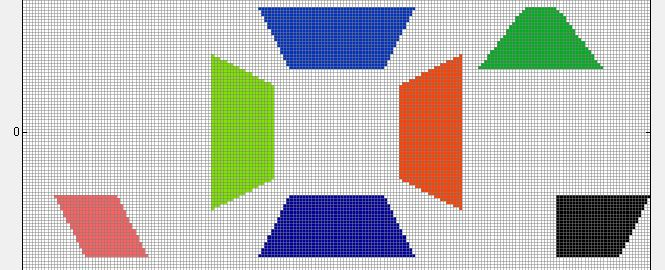Semiellipse

\$regions
region-number      = 1
base-geometry      = semiellipse
region-priority    = 1
semi-ellipse-base  = xmin xmax  ymin ymax
semi-ellipse-top   =    x1         y1
\$end_regions

semi-ellipse-base: Here, 2 values must be equal (meaning only 2 values can be equal), e.g. ymin=ymax=40.0. Then one boundary of the object is a line at y=40 nm. Base plane of the semiellipse must be in a coordinate plane. This plane is identified by the implicit rule, that a pair of coordinate values (e.g.  ymin ymax) has identical values (ymin = ymax).

semi-ellipse-top: This defines a point which determines the height of the semiellipse. In our example (ymin = ymax), the plane is in the (x,z)-coordinate plane. Top coordinates specify an arbitrary point "above" the ellipse, representing the base of the semiellipse.
Top coordinate must be lower than upper coordinate of base line border in direction of axis mentioned above.
Top coordinate must be higher than lower coordinate of base line border in direction of axis mentioned above.
xmin < x1 <xmax
y1 < ymin=ymax
or y1 > ymin=ymax

Examples: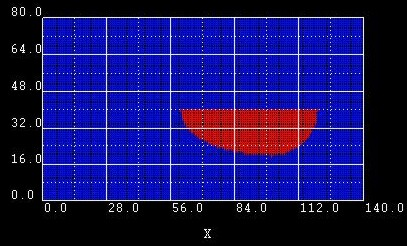semi-ellipse-base  = xmin xmax  ymin ymax  semi-ellipse-top   =    x1         y1  Example 1: semi-ellipse-base =  60.0 120.0  40.0 40.0 semi-ellipse-top  = 100.0        20.0From these data, the following points are extracted:    (point:   (x,y))    base:  ( 60,40) (120,40)    top:   (100,20)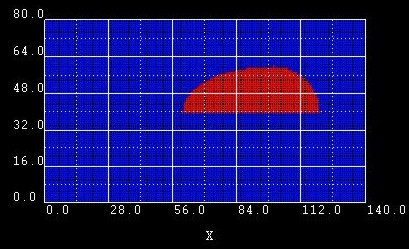Example 2: semi-ellipse-base =  60.0 120.0  40.0 40.0 semi-ellipse-top  = 100.0        60.0 We changed the y1 coordinate of semi-ellipse-top from 20.0 to 60.0.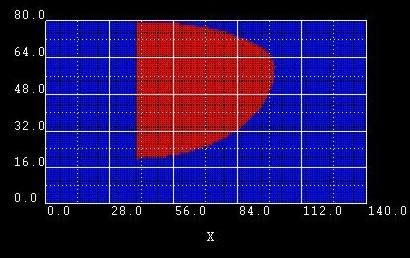Example 3: semi-ellipse-base =  40.0 40.0  20.0 80.0 semi-ellipse-top  = 100.0       60.0 Here we changed semi-ellipse-base: Now the two x coordinates have identical values.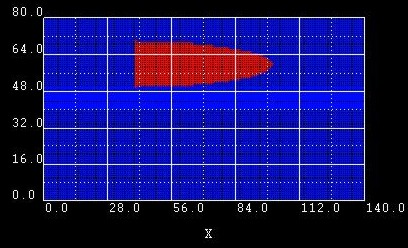Example 4: semi-ellipse-base =  40.0 40.0  50.0 70.0 semi-ellipse-top  = 100.0       60.0 Here we changed the ymin and ymax coordinates of semi-ellipse-base: Now the y extension of the semiellipse is restricted from ymin = 50 nm to ymax = 70 nm. The baseline is at the fixed value for x = 40 nm.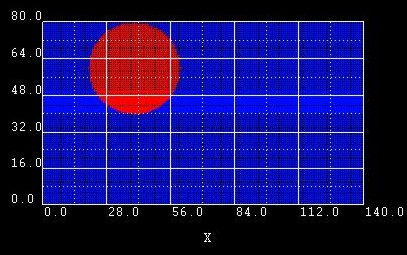Example 5: semi-ellipse-base = 40.0 40.0 40.0 80.0 semi-ellipse-top  = 60.0 60.0 semi-ellipse-base = 40.0 40.0 40.0 80.0 semi-ellipse-top  = 20.0 60.0 Here we built a circle out of 2 semi-ellipses. However, it is obviously easier to use circle instead.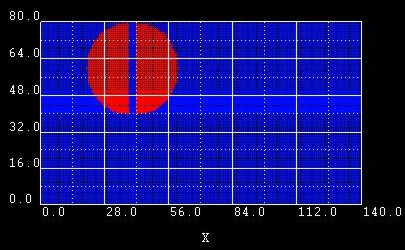Example 6: semi-ellipse-base = 42.0 42.0 40.0 80.0 semi-ellipse-top  = 60.0 60.0 semi-ellipse-base = 38.0 38.0 40.0 80.0 semi-ellipse-top  = 20.0 60.0 Same as example 5 but this time, we moved the baselines a little bit apart from each other to make example 5 easier to understand.

3-dimensional objects

Cuboid

\$regions
region-number     = 1
base-geometry     = cuboid
region-priority   = 1
x-coordinates     = xmin  xmax
y-coordinates     = ymin  ymax
z-coordinates     = zmin  zmax
\$end_regions

The surfaces of the cuboid are assumed to be in coordinate planes of the simulation coordinate system. The coordinates above specify the six coordinate planes which limit the cuboid.

Sphere

\$regions
region-number     = 1
base-geometry     = sphere
region-priority   = 1
center            = x     y     z  ! [nm]
\$end_regions

The sphere is defined by a center with coordinates (x,y,z) and a radius r.

Obelisk

\$regions
region-number      = 1
base-geometry      = obelisk
region-priority    = 1
base-coordinates   = xmin xmax  ymin ymax  zmin zmax
top-coordinates    = xmin xmax  ymin ymax  zmin zmax
\$end_regions

Base and top plane of the obelisk have to be in parallel coordinate planes. These planes are identified by the implicit rule, that a pair of coordinate values (e.g.  ymin ymax) has the same value (ymin = ymax).
In this example, the plane is in the (x,z)-coordinate plane. The remaining four coordinates specify a rectangle in the corresponding plane.

Truncated cone and cylinder

\$regions
region-number      = 1
base-geometry      = truncated-cone                    ! can be used to specify a cylinder
region-priority    = 1
base-coordinates   = xmin xmax  ymin ymax  zmin zmax
top-coordinates    = xmin xmax  ymin ymax  zmin zmax
\$end_regions

A cone with its apex cut off by a plane is called a truncated cone. In our implementation, the truncated cone is bounded by two ellipses of different size that are aligned parallel to each other.

Base and top plane of the truncated cone have to be in parallel coordinate planes. This plane is identified by the implicit rule, that a pair of coordinate values (e.g.  xmin xmax) has the same value (xmin = xmax). In this example, the plane is in the (y,z)-coordinate plane.  ymin ymax  and zmin zmax  specify the diameter of the truncated cone top and base in the y and z direction, respectively. This corresponds to the specification of ellipses in the base and top plane.

How to specify a cylinder?

A cylinder is specified as a special case of a truncated-cone where the two boundary planes are circles. For a truncated cone, one specifies base and top coordinates. Let us assume we have a spherical cylinder of diameter 10 nm and height 15 nm. Then the base and top coordinates would be, for example,
base-coordinates   =
10.0 20.0   10.0 20.0  15.0 15.0   ! (xmin,xmax,ymin,ymax,zmin,zmax) = (10,20,10,20,15,15)
top-coordinates    = 10.0 20.0   10.0 20.0  30.0 30.0   ! (xmin,xmax,ymin,ymax,zmin,zmax) = (10,20,10,20,30,30)
In other words, the x and y-coordinates specify the principal axes of the bottom and top ellipse (or circle) of the cylinder, respectively, and the z-coordinates specify the planes in which these two ellipses lie.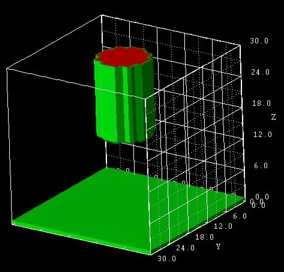Cone

\$regions
region-number      = 1
base-geometry      = cone
region-priority    = 1
base-coordinates   = xmin xmax  ymin ymax  zmin zmax
top-coordinates    = x y z
\$end_regions

Base plane of the cone has to be in parallel to the coordinate system planes. This plane is identified by the implicit rule, that a pair of coordinate values (e.g.  xmin xmax) has the same value (xmin = xmax). In this example, the plane is in the (y,z)-coordinate plane.  ymin ymax  and zmin zmax  specify the diameter of the cone base in the y and z direction, respectively. This corresponds to the specification of an ellipse in the base plane. The top of the cone, the apex, is defined by the point (x,y,z).

The cone does not have to be right circular (where circular means that the base is a circle and right means that the axis passes through the center of the base at right angles to its plane).
Oblique cones are allowed, in which the axis does not pass perpendicularly through the center of the base.

The following figure shows several cones and truncated-cones (including the special case of cylinders). The related input file is called 3D_cone.in.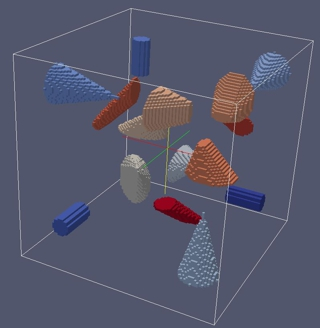The cones are defined as follows:

!-------------------------------------------------------------------------------
! This is a cylinder. The base and top planes are parallel to the (y,z) plane.
!------------------------------------------------------------------------------
region-number    = 1    base-geometry = truncated-cone     region-priority = 1      ! cylinder
base-coordinates =  80.0 80.0     -80.0 -60.0     -80.0 -60.0                       ! xmin xmax ymin ymax zmin zmax
top-coordinates  =  40.0 40.0     -80.0 -60.0     -80.0 -60.0                       ! xmin xmax ymin ymax zmin zmax

!-------------------------------------------------------------------------------
! This is a right circular cone. The base plane is parallel to the (y,z) plane.
!-------------------------------------------------------------------------------
region-number    = 2    base-geometry = cone               region-priority = 1      ! cone (right circular)
base-coordinates = -80.0 -80.0     50.0 90.0     50.0 90.0                          ! xmin xmax ymin ymax zmin zmax
top-coordinates  = -10.0 70.0 70.0                                                  ! x y z

!--------------------------------------------------------------------------------------------------------
! This is a cone where the projection of the apex onto the base plane is located outside the base plane.
! The base plane is parallel to the (y,z) plane.
!--------------------------------------------------------------------------------------------------------
region-number    = 3    base-geometry = cone               region-priority = 2      ! cone
base-coordinates = -10.0 -10.0    -60.0 -20.0    -80.0 -20.0                        ! xmin xmax ymin ymax zmin zmax
top-coordinates  =  40.0 -10.0 -10.0                                                ! x y z

!--------------------------------------------------------------------------
! This is a truncated cone. The base plane is parallel to the (y,z) plane.
!--------------------------------------------------------------------------
region-number    = 4   base-geometry = truncated-cone     region-priority = 3      ! truncated-cone
base-coordinates =  20.0 20.0      50.0 90.0      50.0 100.0                        ! xmin xmax ymin ymax zmin zmax
top-coordinates  =  50.0 50.0      60.0 80.0      60.0  70.0                        ! xmin xmax ymin ymax zmin zmax

!--------------------------------------------------------------------------------------------------------------------
! These are three truncated cones where the projection of the top plane on the base plane is outside the base plane.
! The base plane is parallel to the (y,z) plane.
!--------------------------------------------------------------------------------------------------------------------
region-number    = 5    base-geometry = truncated-cone     region-priority = 4      ! truncated-cone
base-coordinates = -50.0 -50.0     20.0 40.0      20.0  60.0                        ! xmin xmax ymin ymax zmin zmax
top-coordinates  = -20.0 -20.0     50.0 80.0      20.0  40.0                        ! xmin xmax ymin ymax zmin zmax

Semiellipsoid

\$regions
region-number      = 1
base-geometry      = semiellipsoid
region-priority    = 1
base-coordinates   = xmin xmax  ymin ymax  zmin zmax
top-coordinates    = xtop ytop ztop
\$end_regions

Base plane of the semiellipsoid must be in a coordinate plane. This plane is identified by the implicit rule, that a pair of coordinate values (e.g.  ymin ymax) has identical values (ymin = ymax).
In this example, the plane is in the (x,z)-coordinate plane. Top coordinates specify an arbitrary point "above" the ellipse, representing the base of the semiellipsoid.

Example: 3D sphere

A 3D sphere can be constructed from two semiellipsoids. However, it is obviously easier to use sphere instead.
In this example, the bottom planes of the two half-spheres are at z = 5 nm.
The upper half-sphere extends from 5 nm to 6 nm, the lower half-sphere from 5 nm to 4 nm.
The extensions in x and y directions for both half-spheres are from 4 nm to 6 nm.
Consequently, the sphere has a diameter of 2 nm.

region-number    = 1
base-geometry    = semiellipsoid                 !
(upper half-sphere)
base-coordinates = 4.0 6.0   4.0 6.0   5.0 5.0   ! xmin xmax ymin ymax zmin=zmax
top-coordinates  = 5.0 5.0 6.0                   ! xtop ytop ztop

region-number    = 2
base-geometry    = semiellipsoid                 !
(lower half-sphere)
base-coordinates = 4.0 6.0   4.0 6.0   5.0 5.0   ! xmin xmax ymin ymax zmin=zmax
top-coordinates  = 5.0 5.0 4.0                   ! xtop ytop ztop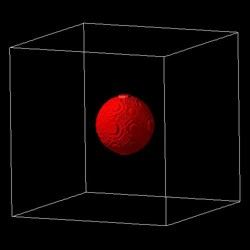Hexagonal-obelisk

\$regions
region-number      = 1
base-geometry      = hexagonal-obelisk
region-priority    = 1
base-coordinates   = xmin xmax  ymin ymax  zmin zmax
top-coordinates    = xmin xmax  ymin ymax  zmin zmax
\$end_regions

Base and top plane of the hexagonal-obelisk have to be in parallel coordinate planes. These planes are identified by the implicit rule, that a pair of coordinate values (e.g.  zmin zmax) has the same value (zmin = zmax). In this example, the plane is in the (x,y)-coordinate plane. The remaining four coordinates specify a rectangle in the corresponding plane.
This geometry element is useful for wurtzite.
Many thanks to Lu Fu-Fa (Institute of Technology (CCIT), Taiwan, R.O.C.) for useful suggestions regarding the implementation of this geometry element.

Two hexagonal-obelisk shapes are possible:

• 'hexagonal-cylinder' with 6-fold rotational symmetry axis oriented along the z direction (i.e. zmin = zmax).
Requirements: xmin(base) = xmin(top)
xmax(base) = xmax(top)
ymin(base) = ymin(top)
ymax(base) = ymax(top)
Note: This condition should be fullfilled: ymax(base) - ymin(base) > (xmax(base) - xmin(base)) / 0.866
• 'hexagonal-pyramid' with 6-fold rotational symmetry axis oriented along the z direction (i.e. zmin = zmax).
Requirements: xmin(top), xmax(top), ymin(top), ymax(top) arbitrary
Note: This condition should be fullfilled: ymax - ymin > (xmax - xmin) / 0.866

For both shapes it holds:

• height of pyramid/cylinder: zmax(top) - zmax(base)                  (zmin = zmax)
• width in x direction (distance between two parallel planes): xmax(base) - xmin(base)
• width in y direction (distance between two corners) if the condition ymax(base) - ymin(base) > (xmax(base) - xmin(base)) / 0.866 is fullfilled:
[xmax(base) - xmin(base)]/0.866 [Note: 0.866 = cos(30°)]
If the above cited condition is not fullfilled, then the width is: ymax(base) - ymin(base)
• width along y > width along x if ymax(base) - ymin(base) > xmax(base) - xmin(base)
• center of hexagonal base plane on x axis: 0.5*(xmin(base) + xmax(base))
• center of hexagonal base plane on y axis: 0.5*(ymin(base) + ymax(base)): ymin(base) and ymax(base) can be used to shift the hexagon along the y axis.

Two sides of the hexagonal base plane are aligned parallel to the y axis.
To rotate the hexagonal base plane by 30 degrees, the user has to specify values for xmin(top), xmax(top), ymin(top), ymax(top) so that it holds:
xmax(top) - xmin(top) > ymax(top) - ymin(top)

In this case it holds:

• width in x direction (distance between two corners) if the condition xmax(base) - xmin(base) > (ymax(base) - ymin(base)) / 0.866 is fullfilled:
[ymax(base) - ymin(base)]/0.866 [Note: 0.866 = cos(30°)]
• width in y direction (distance between two parallel planes): ymax(base) - ymin(base)
• width along y < width along x if xmax(base) - xmin(base) > ymax(base) - ymin(base)
• center of hexagonal base plane on x axis: 0.5*(xmin(base) + xmax(base)): xmin(base) and xmax(base) can be used to shift the hexagon along the x axis.

If the 6-fold rotational axis is oriented along the x (i.e. xmin = xmax) or y directions (i.e. ymin = ymax), cyclic permutations hold for the above statements.

Example input file: 3DHexagonalObelisk.in

Screenshots:

Hexagonal shaped pyramid with flat top plane: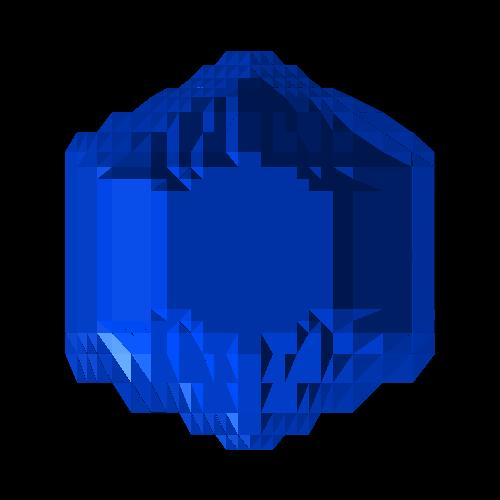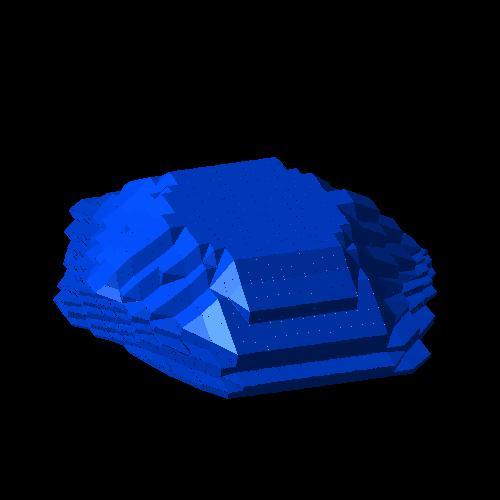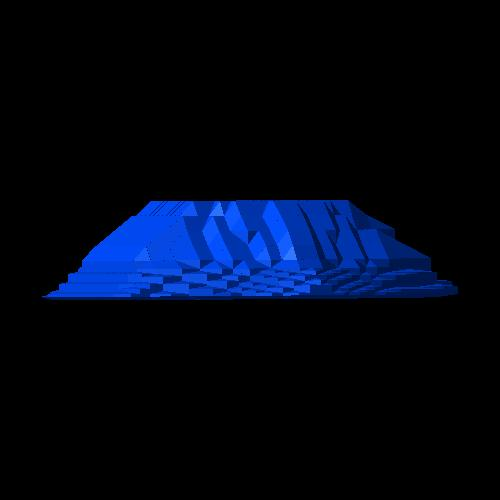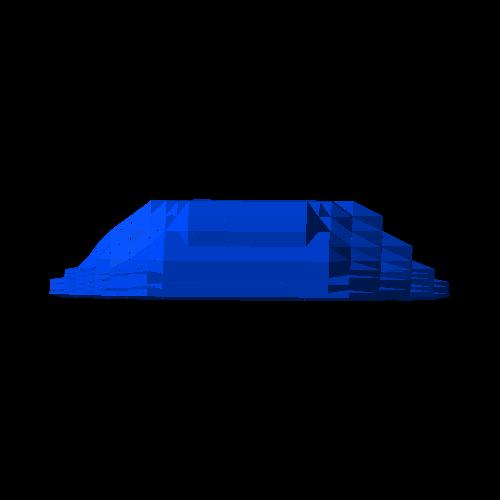Hexagonal shaped pyramid: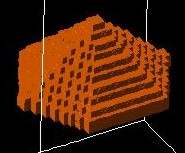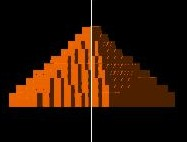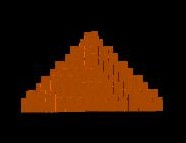Hexagonal shaped "cylinder":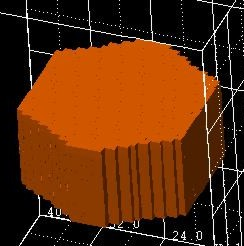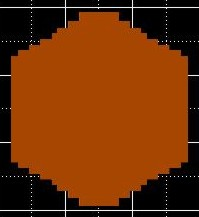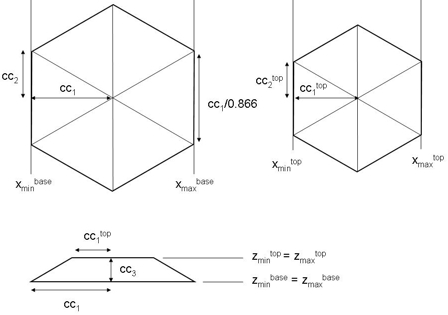Triangular prism

\$regions
region-number      = 1
base-geometry      = triangular-prism
region-priority    = 1
corner-coordinates = x1 y1 z1   x2 y2 z2    x3 y3 z3

x4 y4 z4   x5 y5 z5    x6 y6 z6

\$end_regions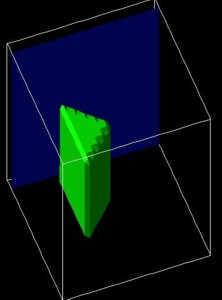Example:
corner-coordinates = 10.0 10.0 10.0 ! x1 y1 z1
10.0 30.0 10.0 ! x2 y2 z2
20.0 20.0 10.0 ! x3 y3 z3

10.0 10.0 40.0 ! x4 y4 z4
10.0 30.0 40.0 ! x5 y5 z5
20.0 20.0 40.0 ! x6 y6 z6

Restrictions: triangular-prism must be oriented so that the triangles are perpendicular to either the x, y or z directions.
Example: Triangles perpendicular to z direction. Then it must hold:
==> corner-coordinates => z1 = z2 = z3
z4 = z5 = z6